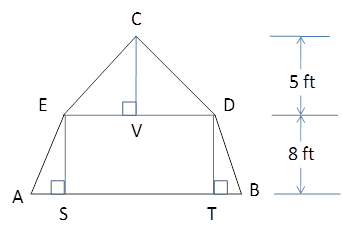Chapter 4.4, Problem 39EElementary Geometry For College St...

7th Edition
Alexander + 2 others
ISBN: 9781337614085

Solutions

Chapter
SectionElementary Geometry For College St...

7th Edition
Alexander + 2 others
ISBN: 9781337614085
Textbook Problem

In a gambrel style roof, the gable end of a barn has the shape of an isosceles trapezoid surmounted by an isosceles triangle. If AE=30 ft and BD=24 ft, find: (a) AS (b) VD (c) CD (d) DETo determine

(a)

To Find:

AS

Explanation

Consider the following diagram.

From the given diagram, we know that BSAEandDTAE.

Therefore, BD=ST.

It is given that BD=24ft.

Then, ST=24ft.

Since, ABDE is an isosceles trapezoid ΔABSΔEDT.

Then, AS=TE

To determine

(b)

To Find:

VD

To determine

(c)

To Find:

CD

To determine

(d)

To Find:

DE

Still sussing out bartleby?

Check out a sample textbook solution.

See a sample solution

The Solution to Your Study Problems

Bartleby provides explanations to thousands of textbook problems written by our experts, many with advanced degrees!

Get Started

A sample of n = 9 scores has X = 108. What is the sample mean?

Essentials of Statistics for The Behavioral Sciences (MindTap Course List)

In Exercises 7-28, perform the indicated operations and simplify each expression. 11. 583(3t+2)+13

Applied Calculus for the Managerial, Life, and Social Sciences: A Brief Approach

With an initial estimate of −1, use Newton’s Method once to estimate a zero of f(x) = 5x2 + 15x + 9.

Study Guide for Stewart's Single Variable Calculus: Early Transcendentals, 8th